﻿ Diagram vector icon stock vector. Illustration of organization - 120755144

# Diagram vector icon.

## Diagram vector icon. filled flat sign for mobile concept and web design. hierarchy scheme simple solid icon. Sitemap symbol, logo illustration. Pixel perfect vector graphics

More similar stock illustrations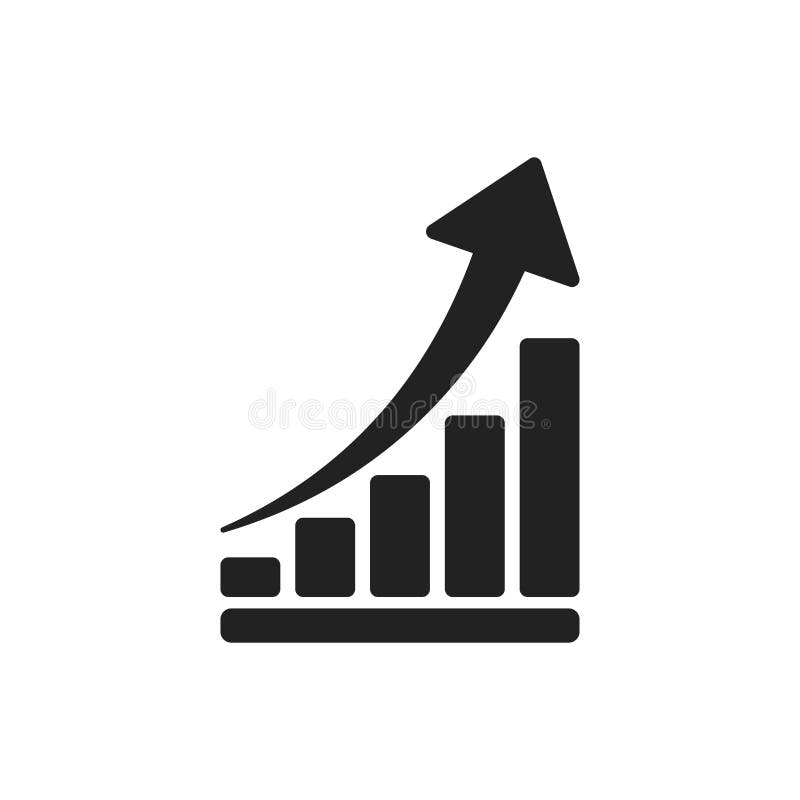Growth chart icon. Grow diagram flat vector illustration. Busine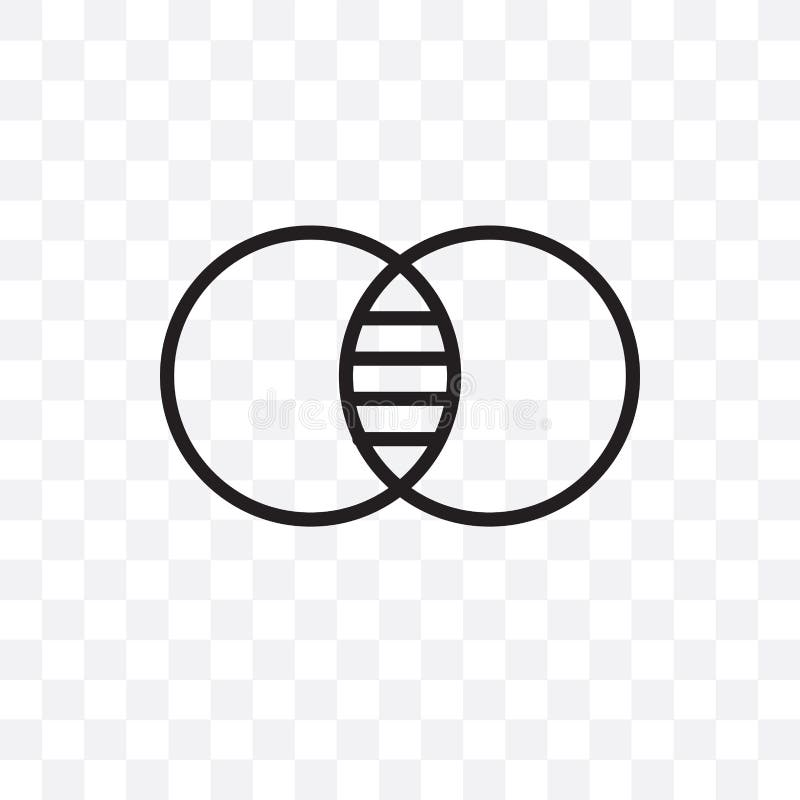Venn diagram vector linear icon isolated on transparent background, Venn diagram transparency concept can be used for web and mobiCluster diagram vector line icon, sign, illustration on background, editable strokesSchematic diagram linear icon concept. Schematic diagram line vector sign, symbol, illustration.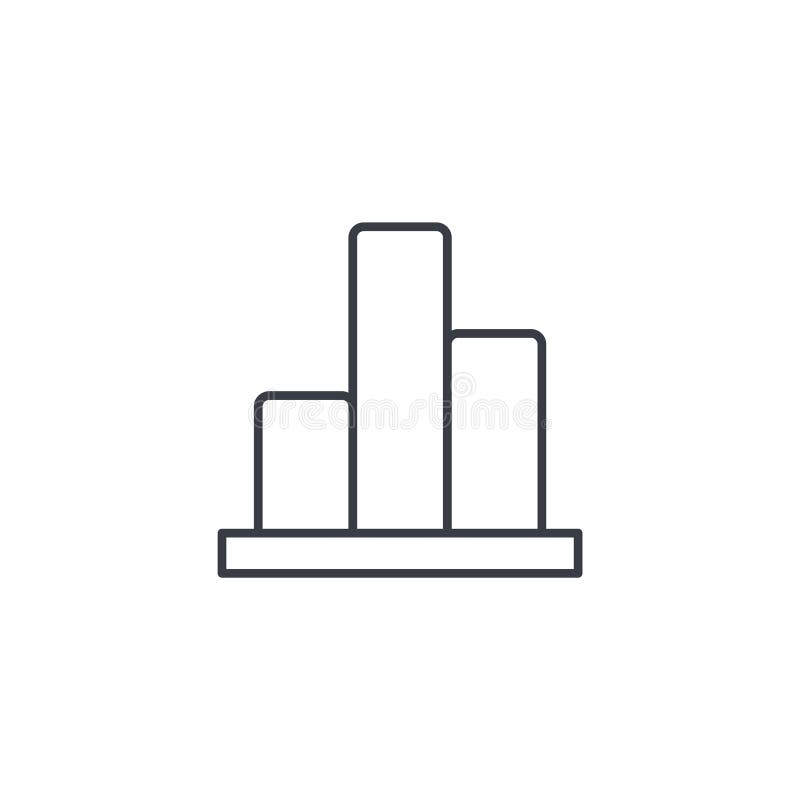Graph chart, statistic diagram thin line icon. Linear vector symbol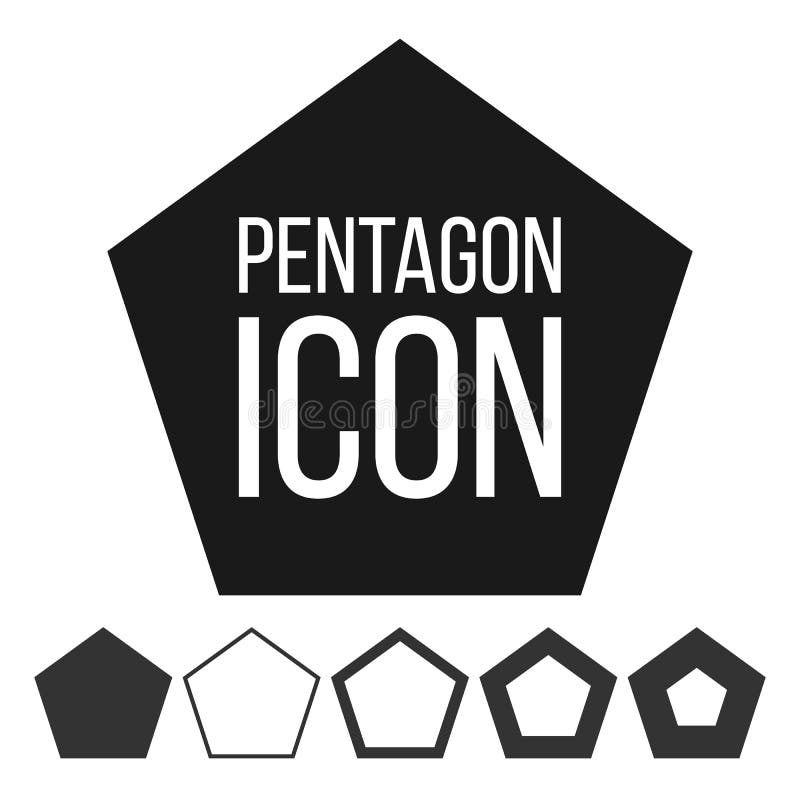Pentagon Icon Vector. 5 Five Sided Symbol. Geometry Chart. Pentagonal Diagram Sign. Polygon Pictogram. Pentagonal IconSWOT Chart strength ,weakesses ,opportunities and threats with icon sign and text sign in block diagram weave Vector illustrati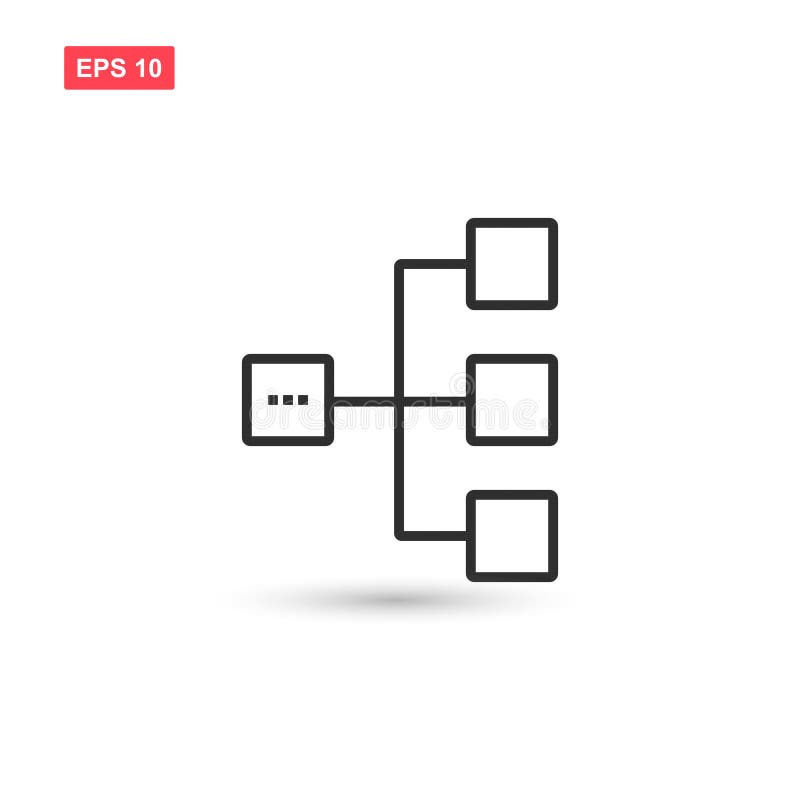Diagram icon vector design isolated 4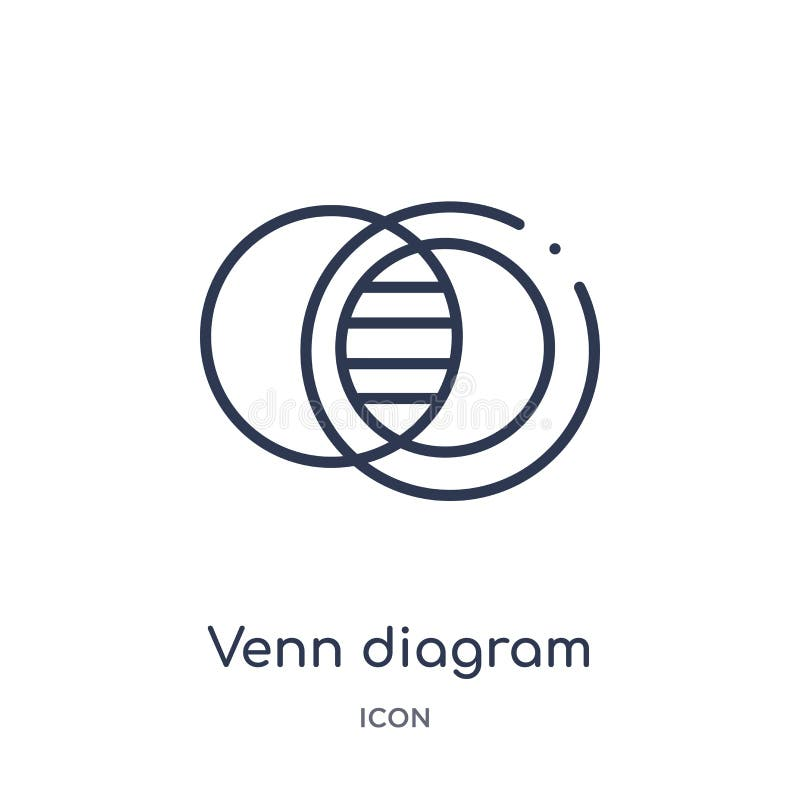Linear venn diagram icon from Analytics outline collection. Thin line venn diagram vector isolated on white background. venn
More stock photos from Aleksey Vanin's portfolio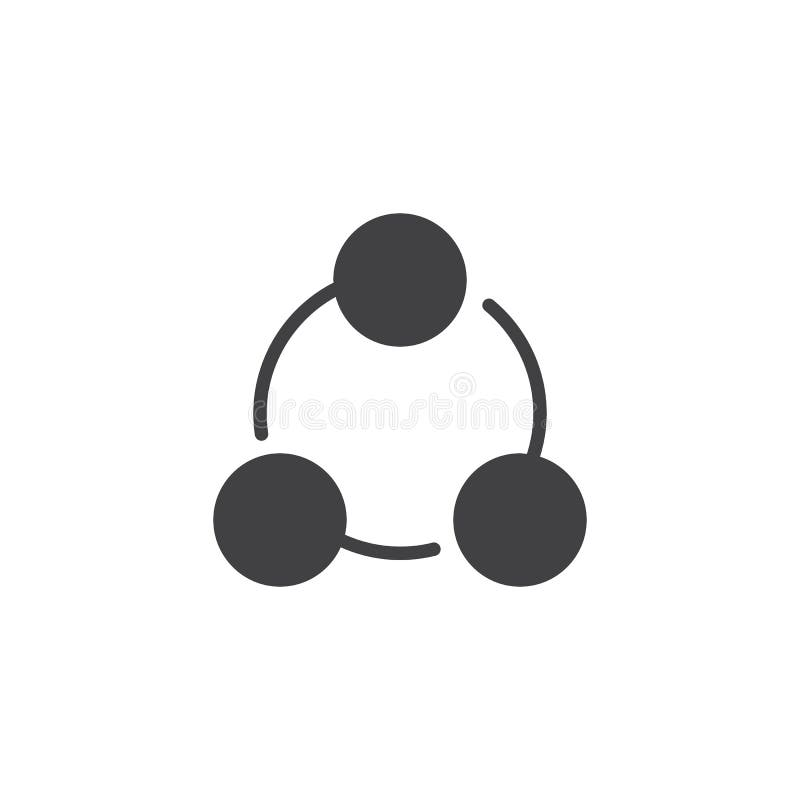Circle diagram vector iconDiagram vector icon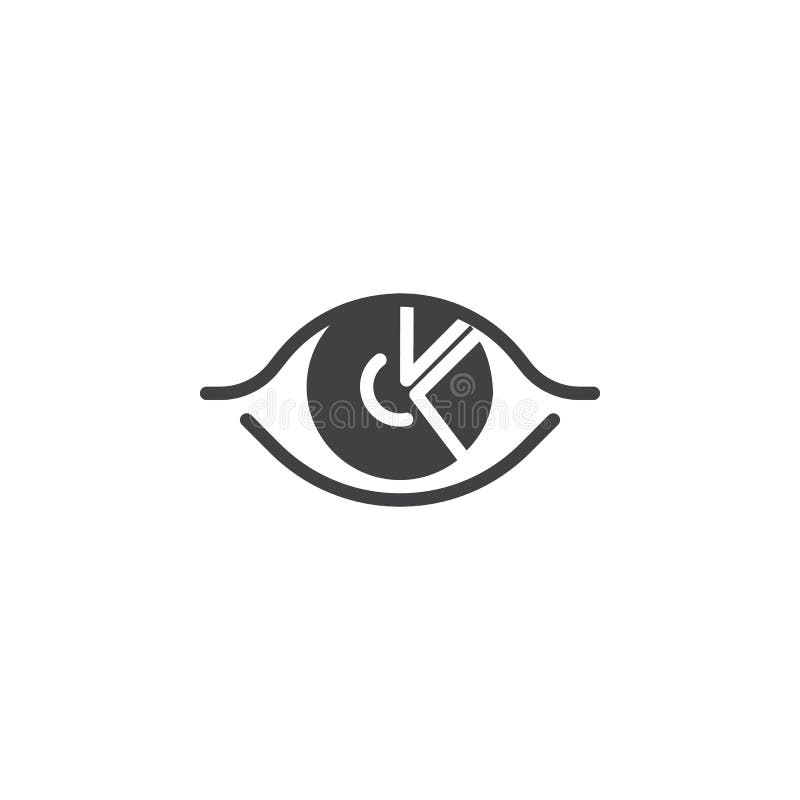Diagram chart analysis vector icon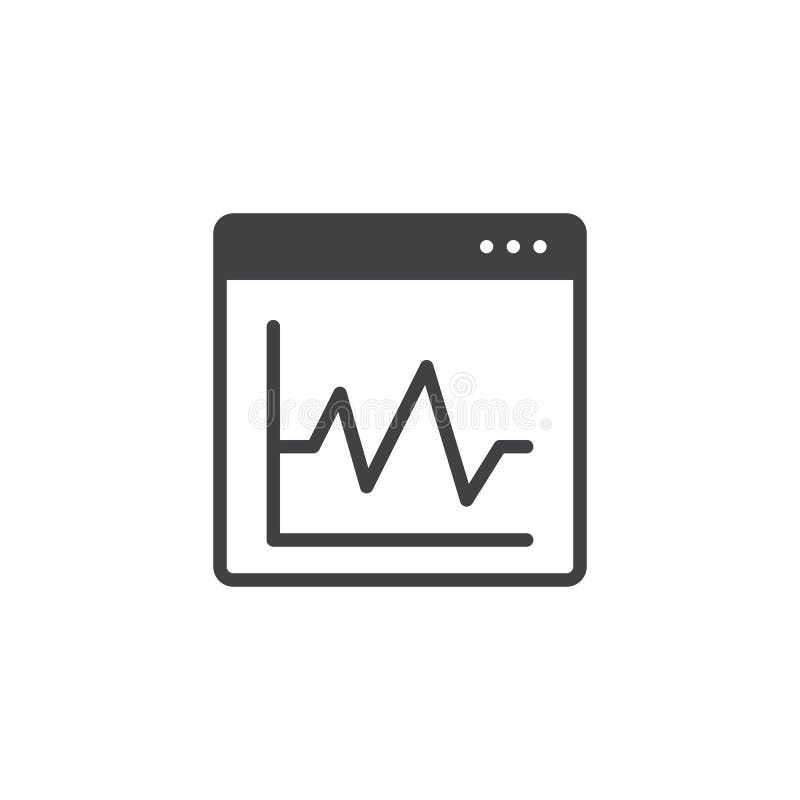Graphic diagram on browser window vector iconDiagram screen vector icon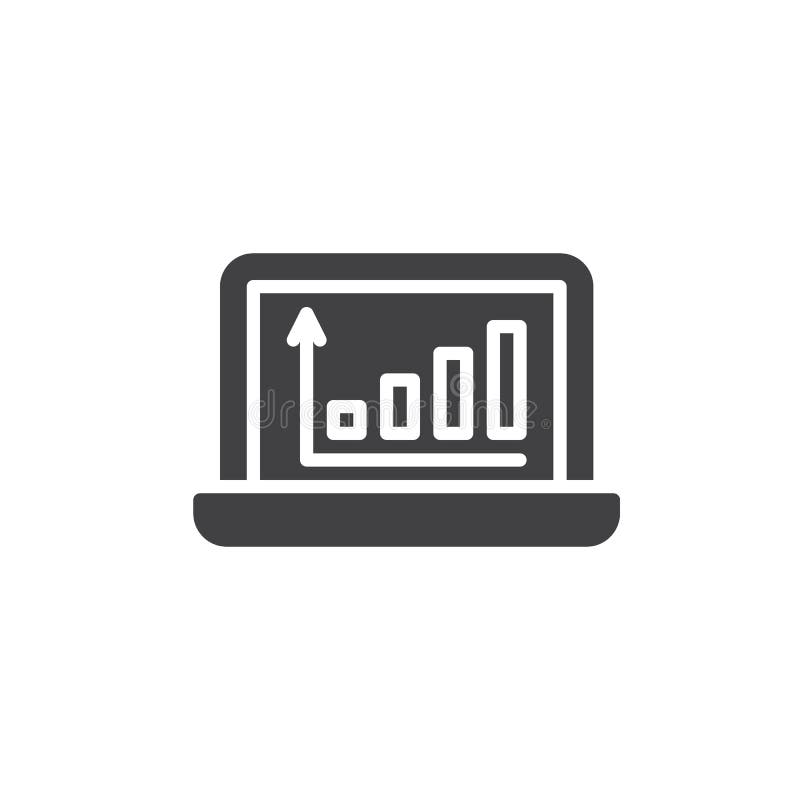Statistics diagram on laptop screen vector icon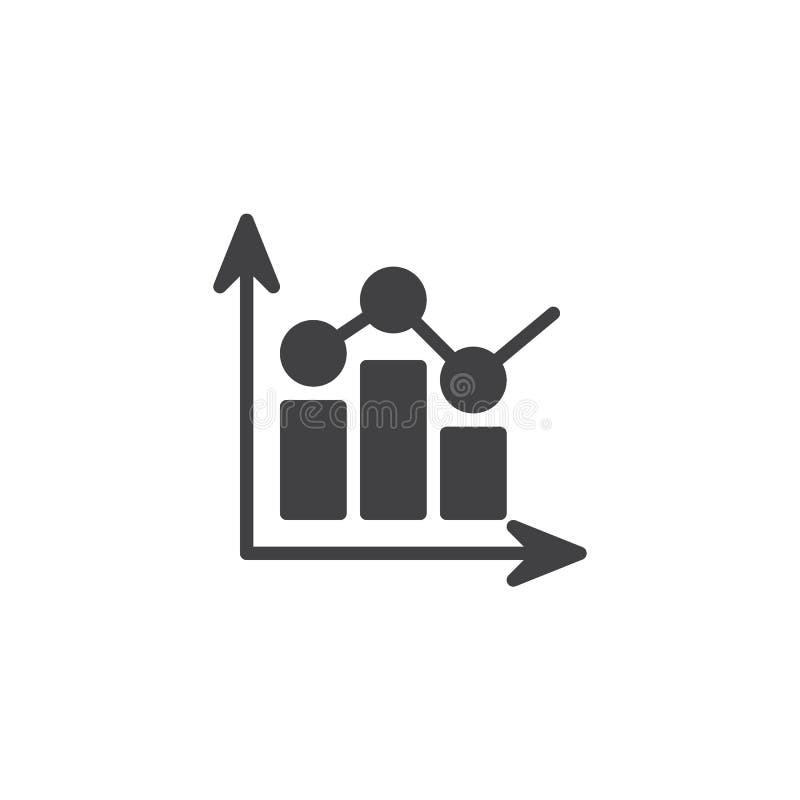Statistics bar diagram vector icon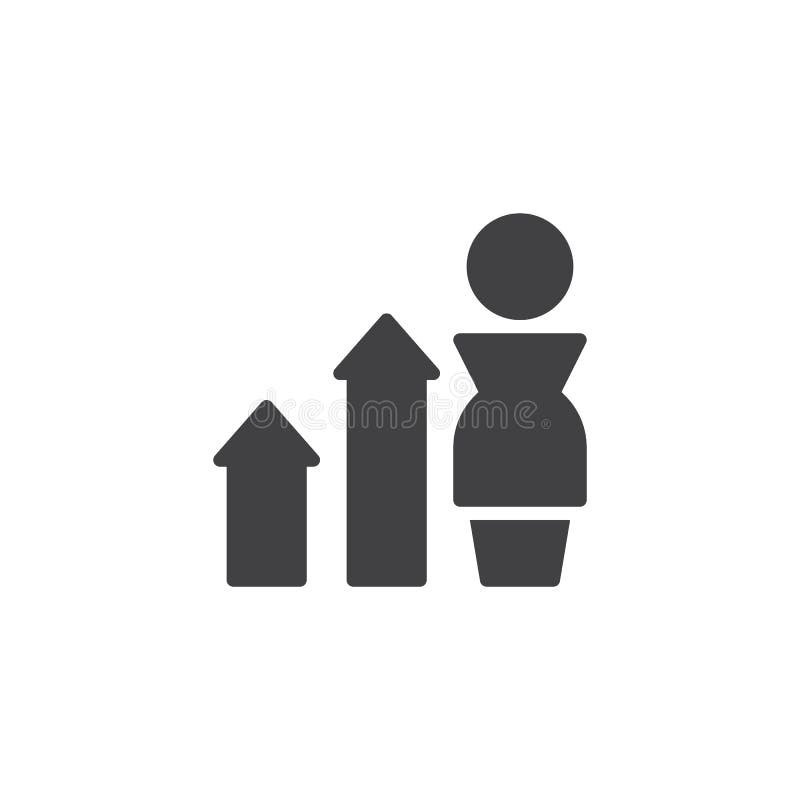Businessman with diagram arrow growth vector iconDiagram clock vector icon
Related categories